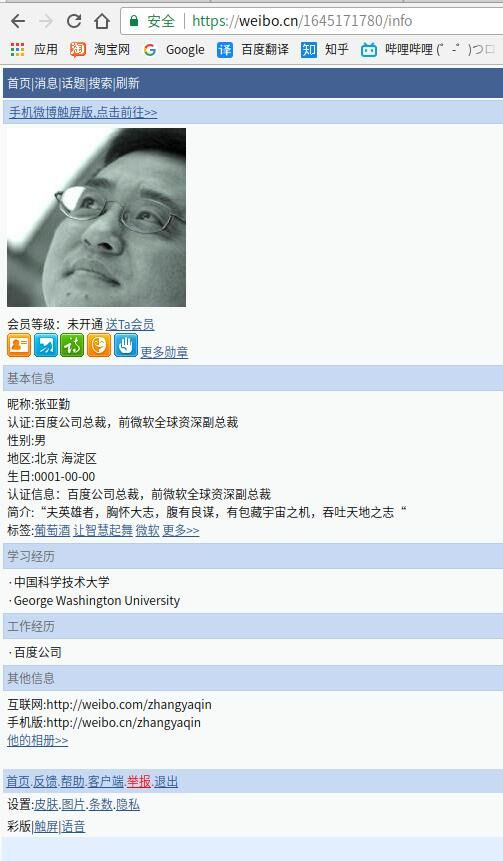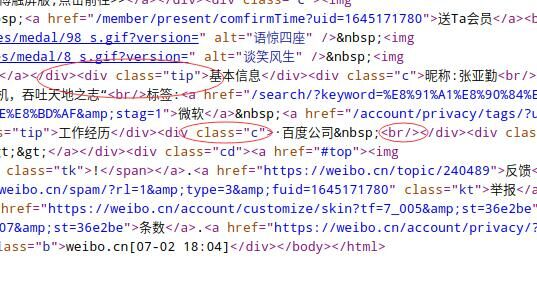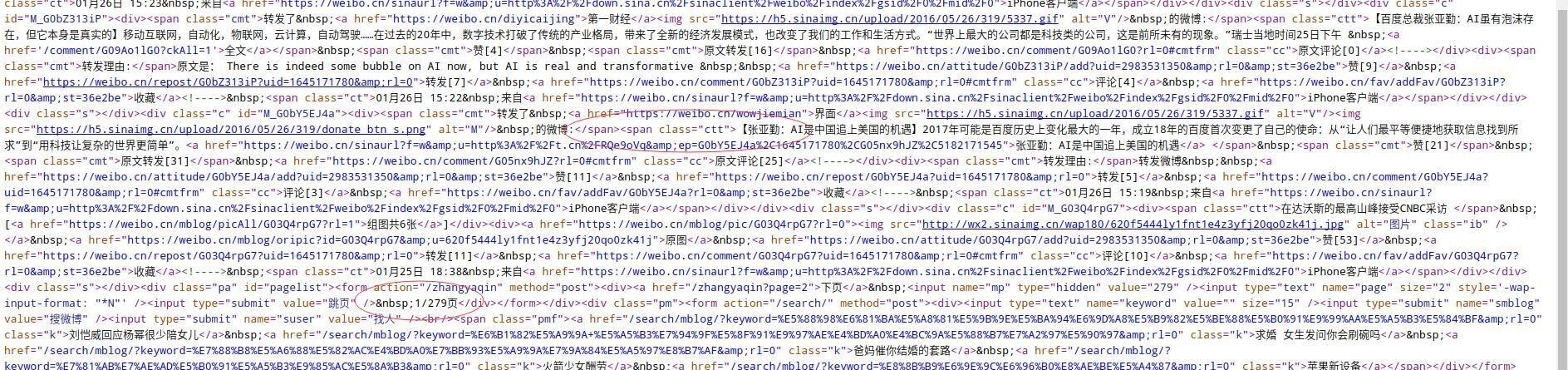Collect from 追梦人物的博客
Modified by ACool

# 爬虫实战（一）：爬取微博用户信息

## 先决条件

• 按F12键打开Chrome开发者工具；
• 点开“Network”，将“Preserve log”选中，输入微博的用户名、密码，登录

### UID## 网页分析

### 用户资料页源码分析```tip = re.compile(r'class="tip">(.*?)></div>', re.S) #匹配四个模块所有内容
title = re.compile(r'(.*?)</div><div', re.S)  # 匹配基本信息/学习经历/工作经历/其他信息
node = re.compile(r'.*?class="c"(.*?)\$', re.S) # 匹配一个模块中的所有内容
info = re.compile(r'>(.*?)<br/', re.S) # 匹配资料条
```

### 用户动态页源码分析```dynamic = re.compile(r'.*?><span class="ctt">(.*?)<a href', re.S)  # 匹配动态
times = re.compile(r'.*?<span class="ct">(.*?)&nbsp', re.S)  # 匹配动态发布时间
```

```page_number = re.compile(r'.*/(\d*?)页</div>', re.S)  # 匹配动态页数
```

## 爬取信息

```tip = re.compile(r'class="tip">(.*?)></div>', re.S) #匹配四个模块所有内容
title = re.compile(r'(.*?)</div><div', re.S)  # 匹配基本信息/学习经历/工作经历/其他信息
node = re.compile(r'.*?class="c"(.*?)\$', re.S) # 匹配一个模块中的所有内容
info = re.compile(r'>(.*?)<br/', re.S) # 匹配资料条
Uname = ''
Certified = ''
Sex = ''
Relationship = ''
Area = ''
Birthday = ''
Education_info = ''
Work_info = ''
Description = ''
for one in tips:
titleone = re.findall(title, one)  # 信息标题

node_tmp = re.findall(node, one)
infos = re.findall(info, node_tmp)  # 信息
if (titleone == '基本信息'):
for inf in infos:
if (inf.startswith('昵称')):
_, Uname = inf.split(':', 1)
elif (inf.startswith('认证信息')):
print(inf)
_, Certified = inf.split('：', 1)
elif (inf.startswith('性别')):
_, Sex = inf.split(':', 1)
elif (inf.startswith('感情状况')):
_, Relationship = inf.split(':', 1)
elif (inf.startswith('地区')):
_, Area = inf.split(':', 1)
elif (inf.startswith('生日')):
_, Birthday = inf.split(':', 1)
elif (inf.startswith('简介')):
print(inf.split(':'))
_, Description = inf.split(':', 1)
else:
pass
elif (titleone == '学习经历'):
for inf in infos:
Education_info += inf.strip('·').replace("&nbsp", '') + " "
elif (titleone == '工作经历'):
for inf in infos:
Work_info += inf.strip('·').replace("&nbsp", '') + " "
else:
pass
```

```dynamic = re.compile(r'.*?><span class="ctt">(.*?)<a href', re.S)  # 匹配动态
times = re.compile(r'.*?<span class="ct">(.*?)&nbsp', re.S)  # 匹配动态发布时间
page_number = re.compile(r'.*/(\d*?)页</div>', re.S)  # 匹配动态页数
dys = re.findall(dynamic, res.text)
ts = re.findall(times, res.text)
pages = re.findall(page_number, res.text)
pagenums = pages

mainurl = url
label = 0  # 标签用于计数，每5~20次延时10S
tag = random.randint(5, 20)
for pagenum in range(int(pagenums))[1:]:
if (label == tag):
time.sleep(10)
label = 0
tag = random.randint(5, 20)
# 随机选择，防止被ban
'User_Agent': random.choice(user_agents)
}
pagenum += 1
label += 1
url = mainurl + '?page=' + str(pagenum)#更改页数
dys += re.findall(dynamic, page.text)
ts += re.findall(times, page.text)
dys = dys[1:]
```

## 保存数据到数据库

```# 微博用户信息表
Column('user_ID', Integer, primary_key=True, autoincrement=True),  # 主键，自动添加
Column("uid", String(20), unique=True, nullable=False),  # 微博用户的uid
Column("Uname", String(50), nullable=False),  # 昵称
Column("Certified", String(50), default='', server_default=''),  # 认证信息
Column("Sex", String(200), default='', server_default=''),  # 性别nullable=False
Column("Relationship", String(20), default='', server_default=''),  # 感情状况
Column("Area", String(500), default='', server_default=''),  # 地区
Column("Birthday", String(50), default='', server_default=''),  # 生日
Column("Education_info", String(300), default='', server_default=''),  # 学习经历
Column("Work_info", String(300), default='', server_default=''),  # 工作经历
Column("Description", String(2500), default='', server_default=''),  # 简介
mysql_charset='utf8mb4'
)

# 微博用户动态表
Column('data_ID', Integer, primary_key=True, autoincrement=True),  # 主键，自动添加
Column('uid', String(20), ForeignKey(wb_user.c.uid), nullable=False),  # 外键
Column('weibo_cont', TEXT, default=''),  # 微博内容
Column('create_time', String(200), unique=True),  # 创建时间,unique用来执行upsert操作，判断冲突
mysql_charset='utf8mb4'
)

# 动态主题表
Column('topic_ID', Integer, primary_key=True, autoincrement=True),  # 主键，自动添加
Column('uid', String(20), ForeignKey(wb_user.c.uid), nullable=False),  # 外键
Column('topic', Integer, nullable=False),  # 主题-----默认5类
Column('topic_cont', String(20), nullable=False, unique=True),  # 主题内容
mysql_charset='utf8mb4'
)
```

MySQL数据库版本>=5.5.3

MySQL-python 版本 >= 1.2.5

```from sqlalchemy import MetaData, Table
from sqlalchemy.dialects.mysql import insert
ins = insert(table).values(uid=uid, Uname=Uname, Certified=Certified, Sex=Sex, Relationship=Relationship,Area=Area,Birthday=Birthday,Education_info=Education_info,Work_info=Work_info,Description=Description)
ins = ins.on_duplicate_key_update(
# 如果不存在则插入，存在则更新(upsert操作#http://docs.sqlalchemy.org/en/latest/dialects/mysql.html#mysql-insert-on-duplicate-key-#update)
Uname=Uname, Certified=Certified, Sex=Sex, Relationship=Relationship, Area=Area,
Birthday=Birthday, Education_info=Education_info, Work_info=Work_info, Description=Description
)
conn.execute(ins)
```

```re_nbsp = re.compile(r'&nbsp', re.S)  # 去除\$nbsp
re_html = re.compile(r'</?\w+[^>]*>', re.S)  # 去除html标签
re_200b = re.compile(r'\u200b', re.S)  # 去除分隔符
re_quot = re.compile(r'&quot', re.S)
for i in range(len(ts)):#len(ts)为动态数
#去除噪声
dys[i] = re_nbsp.sub('', dys[i])
dys[i] = re_html.sub('', dys[i])
dys[i] = re_200b.sub('', dys[i])
dys[i] = re_quot.sub('', dys[i])
ins = insert(table).values(uid=uid, weibo_cont=pymysql.escape_string(dys[i]), create_time=ts[i])
ins = ins.on_duplicate_key_update(weibo_cont=pymysql.escape_string(dys[i]))
conn.execute(ins)
```